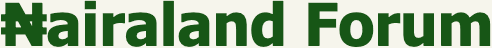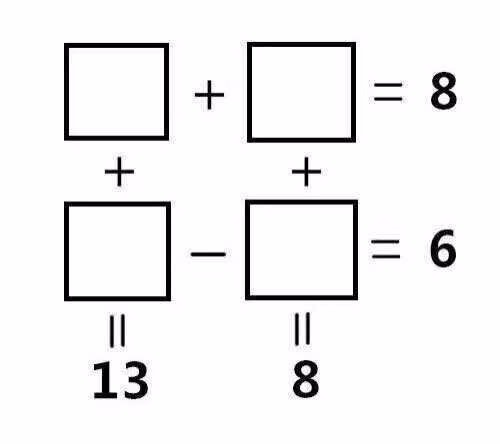#Welcome, Guest: Join Nairaland / LOGIN! / Trending / Recent / New
Stats: 2,868,543 members, 6,884,469 topics. Date: Friday, 27 May 2022 at 08:31 PM

## If You Can Solve These Math Problems, You Are One Of The World's Smartest - Education - Nairaland

 If You Can Solve These Math Problems, You Are One Of The World's Smartest by Bashkhan: 11:21am On Dec 18, 2016 Let see who will get it correctRe: If You Can Solve These Math Problems, You Are One Of The World's Smartest by AndreRose(f): 11:23am On Dec 18, 2016 hmmm Re: If You Can Solve These Math Problems, You Are One Of The World's Smartest by ademasta(m): 11:36am On Dec 18, 2016 So cheap! (3.5)+(4.5)=8 + +(9.5)-(3.5)=6 ^ ^13 8Simple Re: If You Can Solve These Math Problems, You Are One Of The World's Smartest by Kendzyma(m): 3:34pm On Dec 18, 2016 Bashkhan:Let see who will get it correct W + X=8 + + Y - Z=6 II II 13 8W+X=8..........1Y-Z=6............2W+Y=13..........3X+Z= 8 ...........4Solving the four equations ,u should getW= 3.5X=4.5Y=9.5Z=3.5 Re: If You Can Solve These Math Problems, You Are One Of The World's Smartest by Oluwatosint(m): 10:53pm On Dec 18, 2016 Kendzyma: W + X=8 + + Y - Z=6 II II 13 8 W+X=8..........1 Y-Z=6............2 W+Y=13..........3 X+Z= 8 ...........4 Solving the four equations ,u should get W= 3.5 X=4.5 Y=9.5 Z=3.5 Help Solve pleaseRe: If You Can Solve These Math Problems, You Are One Of The World's Smartest by Oluwatosint(m): 11:47pm On Dec 18, 2016 please help solve